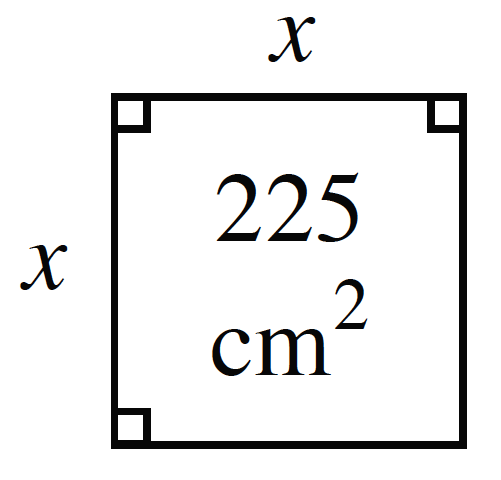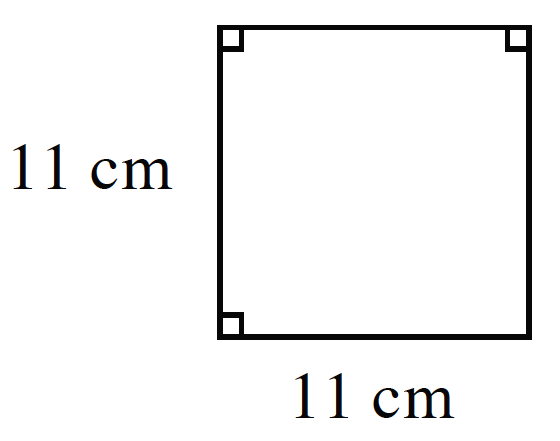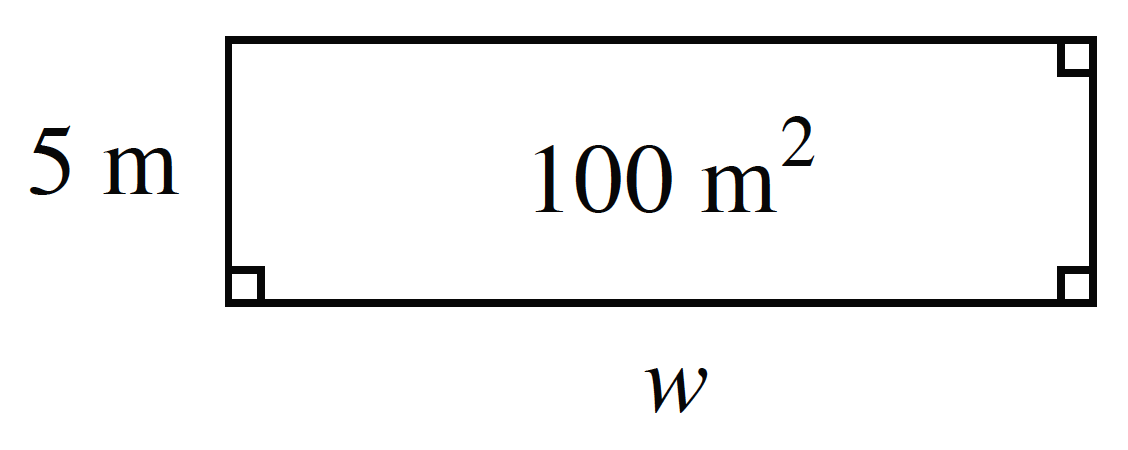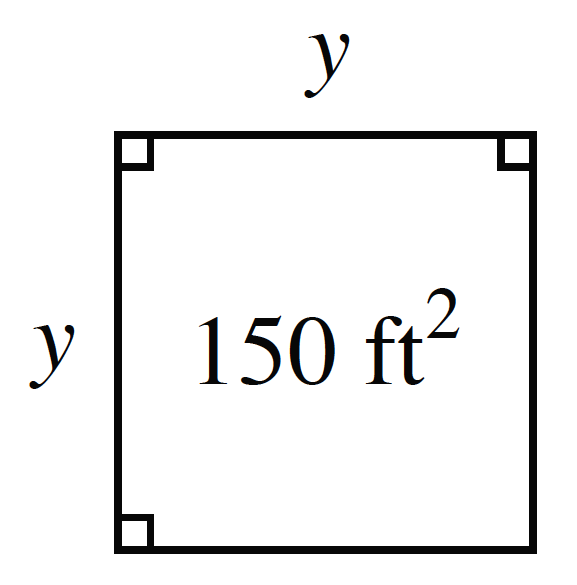### Home > ACC7 > Chapter 9 Unit 10 > Lesson CC3: 9.2.3 > Problem9-88

9-88.

Find the missing length or area.

1.You can identify this figure as a square, which means all the side lengths have equal values.

$x^{2} = 225$
Solve for $x$.

$x=\sqrt{(225)}$

1.$\text{Area = (side)}^{2}$

$121 \text{ cm}^{2}$

1.$\text{Area = length (width)}$

$100 = 5w$
Solve for $w$.

1.See (a).